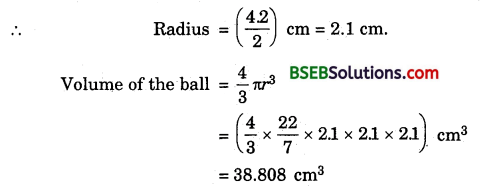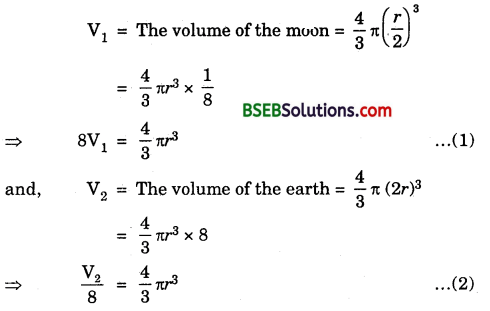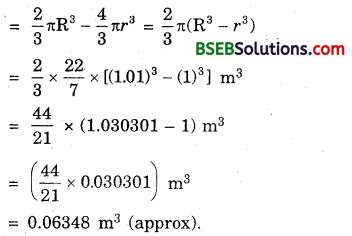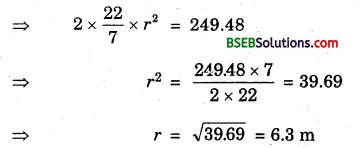# Bihar Board Class 9th Maths Solutions Chapter 13 Surface Areas and Volumes Ex 13.8

Bihar Board Class 9th Maths Solutions Chapter 13 Surface Areas and Volumes Ex 13.8 Textbook Questions and Answers.

## BSEB Bihar Board Class 9th Maths Solutions Chapter 13 Surface Areas and Volumes Ex 13.8Question 1.
Find the volume of a sphere whose radius is
(i) 7 cm
(ii) 0.63 m
Solution:
(i) We have : r = radius of the sphere = 7 cm.(ii) We have : r = radius of the sphere = 0.63 mQuestion 2.
Find the amount of water displaced by a solid spherical ball of diameter
(i) 28 cm
(ii) 0.21 m
Solution:
(i) Diameter of the spherical ball = 28 cm
∴ Radius = $$\frac { 28 }{ 2 }$$ cm = 14 cm
Amount of water displaced by the spherical ball
= Its volume = $$\frac { 4 }{ 3 }$$ πr³
= ($$\frac { 4 }{ 3 }$$ x $$\frac { 22 }{ 7 }$$ x 14 x 14 x 14) cm³
= $$\frac { 34496 }{ 3 }$$ cm³
= 11498$$\frac { 2 }{ 3 }$$ cm³
= 11498$$\frac { 2 }{ 3 }$$ cm³.

(ii) Diameter of the spherical ball = 0.21 m
∴ Radius = ($$\frac { 0.21 }{ 2 }$$) = 0.105 m
Amount of water displaced by the spherical ball
= Its volume = $$\frac { 4 }{ 3 }$$ πr³
= ($$\frac { 4 }{ 3 }$$ x $$\frac { 22 }{ 7 }$$ x 0.105 x 0105 x 0105) m³
= 0.004851 m³.Question 3.
The diameter of a metallic ball is 4.2 cm. What is the mass of the ball, if the density of the metal is 8.9 g per cm³?
Solution:
Diameter of the ball = 4.2 cmDensity of the metal is 8.9 g per cm³
Mass of the ball = (38.808 x 8.9) g = 345.3912 g.

Question 4.
The diameter of the moon is approximately one- fourth the diameter of the earth. What fraction of the volume of the earth is the volume of the moon?
Solution:
Let the diameter of the moon be r. Then, the radius of the moon = $$\frac { r }{ 2 }$$
According to the question, diameter of the earth is 4r, so 4 r its radius = $$\frac { 4r }{ 2 }$$ = 2rHence, the volume of the earth is $$\frac { 1 }{ 64 }$$ of the volume of the earth.Question 5.
How many litres of milk can a hemispherical bowl of diameter 10.5 cm hold?
Solution:
Diameter of a hemispherical bowl = 10.5 cm.Hence, the hemispherical bowl can hold 303 l (approx.) of milk.

Question 6.
A hemispherical tank is made up of an iron sheet 1 cm thick. If the inner radius is 1 m, then find the volume of the iron used to make the tank.
Solution:
Let R cm and r cm be respectively the external and internal radii of the hemispherical vessel. Then,
R = 1.01 (as thickness = 1 cm = .01 m)
and r = 1 m.
Volume of iron used = External volume – Internal volumeQuestion 7.
Find the volume of a sphere whose surface area is 154 cm².
Solution:
Let r cm be the radius of the sphere.
So, surface area = 154 cm²Question 8.
A dome of a building is in the form of a hemisphere. From inside, it was white-washed at the cost of Rs 498.96. If the cost of white-washing is Rs 2.00 per square metre, find the
(i) inside surface area of the dome.
(ii) volume of the air inside the dome.
Solution:
(i) Inside surface area of the dome(ii) Let r be the radius of the dome.
∴ Surface area = 2πr²Volume of the air inside the dome = Volume of the dome
= $$\frac { 2 }{ 3 }$$ πr³
= $$\frac { 2 }{ 3 }$$ x $$\frac { 22 }{ 7 }$$ x 6.3 x 6.3 x 6.3 m³
= 523.9 m³ (approx).Question 9.
Twenty seven solid iron spheres, each of radius r and surface area S are melted to form a sphere with surface area S’, Find the
(i) radius r’ of the new sphere,
(ii) ratio of S and S’.
Solution:
(i) Volume of 27 solid sphere of radius
r = 27 x $$\frac { 4 }{ 3 }$$πr³ … (1)
Volume of the new sphere of radius r’ = $$\frac { 4 }{ 3 }$$ πr’³ … (2)
According to the problem, we have
$$\frac { 4 }{ 3 }$$πr’³ = 27 x $$\frac { 4 }{ 3 }$$πr³
⇒ r’3 = 27r³ = (3r)³
∴ r’ = 3r.

(ii) Required ratio = $$\frac { S }{ S’ }$$ = $$\frac{4 \pi r^{2}}{4 \pi r^{22}}$$ = $$\frac{r^{2}}{(3 r)^{2}}$$
= $$\frac{r^{2}}{9 r^{2}}$$ = $$\frac { 1 }{ 9 }$$ = 1 : 9.

Question 10.
A capsule of medicine is in the shape of a sphere of diameter 3.5 mm. How much medicine (in mm³) is needed to fill this capsule?
Solution:
Diameter of the spherical capsule = 3.5 mm
Radius = $$\frac { 3.5 }{ 3 }$$ mm
= 1.75 mm.
Medicine needed for its filling = Volume of spherical capsule
= $$\frac { 4 }{ 3 }$$πr³
= ($$\frac { 4 }{ 3 }$$ x $$\frac { 22 }{ 7 }$$ x 1.75 x 1.75 x 1.75) m³
= 22.46 m³ (approx).